# Marine Distributed Radar Signal Identification and Classification Based on Deep Learning

Marine Distributed Radar Signal Identification and Classification Based on Deep Learning

Chang Liu Ruslan Antypenko Iryna Sushko Oksana Zakharchenko Ji Wang

Institute of Electronics and Information Engineering, Guangdong Ocean University, Zhanjiang 524088, China

Research Center of Guangdong Smart Oceans Sensor Networks and Equipment Engineering, Zhanjiang 524088, China

Radio Engineering Faculty, National Technical University of Ukraine ‘Igor Sikorsky Kyiv Polytechnic Institute’, Kyiv 03056, Ukraine

Corresponding Author Email:
wangji@gdou.edu.cn
Page:
1541-1548
|
DOI:
https://doi.org/10.18280/ts.380531
12 July 2021
|
Accepted:
6 September 2021
|
Published:
31 October 2021
| Citation

OPEN ACCESS

Abstract:

Distributed radar is applied extensively in marine environment monitoring. In the early days, the radar signals are identified inefficiently by operators. It is promising to replace manual radar signal identification with machine learning technique. However, the existing deep learning neural networks for radar signal identification consume a long time, owing to autonomous learning. Besides, the training of such networks requires lots of reliable time-frequency features of radar signals. This paper mainly analyzes the identification and classification of marine distributed radar signals with an improved deep neural network. Firstly, the time frequency features were extracted from signals based on short-time Fourier transform (STFT) theory. Then, a target detection algorithm was proposed, which weighs and fuses the heterogenous marine distributed radar signals, and four methods were provided for weight calculation. After that, the frequency-domain priori model feature assistive training was introduced to train the traditional deep convolutional neural network (DCNN), producing a CNN with feature splicing operation. The features of time- and frequency-domain signals were combined, laying the basis for radar signal classification. Our model was proved effective through experiments.

Keywords:

1. Introduction

The existing deep learning neural networks for radar signal identification consume a long time, owing to autonomous learning. Besides, the training of such networks requires lots of reliable time-frequency features of radar signals. To solve these problems, this paper proposes an identification scheme that combines the time- and frequency-domain features of radar signals, and relies on an improved deep neural network to recognize and classify marine distributed radar signals. The main contents and innovations are as follows:

(1) The single and multi- pulse signals in each symbol period were converted into the  corresponding time-frequency images, and the time-frequency features were extracted through short-time Fourier transform (STFT); (2) A target detection algorithm was proposed, which weighs and fuses the heterogenous marine distributed radar signals, and four methods were provided for weight calculation; (3) The frequency-domain priori model feature assistive training was introduced to train the traditional deep CNN (DCNN), and the features of time- and frequency-domain signals were combined as the basis for radar signal classification, producing a CNN with feature splicing operation. The effectiveness of our model was proved through experiments.

2. Time-Frequency Feature Extraction

This paper mainly studies the single and multi-pulse signals of marine distributed radars affected by Gaussian white noise. The communication system is composed of multiple radars connected by the communication link. Let o1(p), o2(p), ...on(p) be the original echo signals received and transmitted by each radar in the distributed radar system; m(p) be the additive Gaussian white noise. Then, the signals at the receiving end of each local radar station can be modeled as:

$e\left( p \right)={{o}_{1}}\left( p \right)+{{o}_{2}}\left( p \right)+...+{{o}_{m}}\left( p \right)+m\left( p \right)$     (1)

According to the theory on the recognition of marine distributed radar signals, the key and fundamental link is how to effectively extract the features of the signals received by each radar station. In the time domain and frequency domain, the form of received signals varies with local radar stations. Based on Fourier transform, feature extraction aims to extract the different features in the time and frequency domains. Since the received signals at radar stations are periodic and cyclo-stationary, this paper adopts the time-frequency feature extraction method of the STFT theory to convert the single and multi-pulse signals in each symbol period into corresponding time-frequency images.

The concept of local spectrum assumes that the signals received by radar stations are stable, if intercepted by a short time window function. Incorporating this concept, the STFT performs Fourier transform on the stable received signals, slides the window function along the time axis, and thus obtain a time-variation image about an entire segment of the received signals in the frequency domain.

Let h(p) be a very short time window function; * be complex conjugate. When h(p)=1 and ∀p, the STFT is essentially the traditional Fourier transform. For continuous signals o(p) received by radar stations, the continuous STFT can be defined as:

$DS{{F}_{o}}\left( p,g \right)=\int_{-\infty }^{\infty }{\left[ o\left( v \right)h*\left( v-p \right) \right]}{{r}^{-j2\pi gv}}dv$    (2)

The inverse of the continuous STFT (2) can be given by:

$o\left( p \right)=\int_{-\infty }^{\infty }{\int_{-\infty }^{\infty }{DS{{F}_{o}}\left( p,g \right)}}h\left( v-p \right){{r}^{-j2\pi gv}}dpdv$    (3)

The continuous STFT has several basic properties: linear time-frequency representation and frequency shift invariance. The latter property can be expressed as:

$\tilde{o}\left( t \right)=o\left( p \right){{r}^{j2\pi g\delta }}\to DS{{F}_{{\tilde{o}}}}\left( p,g \right)=DS{{F}_{o}}\left( p,g-{{g}_{0}} \right)$     (4)

This property can be derived by:

\begin{align} & DS{{F}_{{\tilde{o}}}}\left( p,g \right)=\int_{-\infty }^{\infty }{\left[ \tilde{o}\left( v \right)h*\left( v-p \right) \right]{{r}^{-j2\pi jv}}dv} \\ & =\int_{-\infty }^{\infty }{\left[ o\left( v \right){{r}^{j2\pi gv}}h*\left( v-p \right){{r}^{-j2\pi g{{v}_{0}}}} \right]dv} \\ & =\int_{-\infty }^{\infty }{\left[ o\left( v \right)h*\left( v-p \right) \right]{{r}^{-j2\pi \left( g-{{g}_{0}} \right)v}}dv} \\ & =DS{{F}_{o}}\left( p,g-{{g}_{0}} \right) \\\end{align}      (5)

The time shift invariance can be expressed as:

\begin{align} & \tilde{o}\left( p \right)=o\left( p-{{p}_{0}} \right)\to \\ & DS{{F}_{{\tilde{o}}}}\left( p,g \right)=DS{{F}_{o}}\left( p-{{p}_{0}},g \right){{r}^{-j2\pi {{p}_{0}}g}} \\\end{align}     (6)

That is, DSFo~(p,g)=DSFo(p-p0,g) does not hold. This property can be derived by:

\begin{align} & DS{{F}_{{\tilde{o}}}}\left( p,g \right)=\int_{-\infty }^{\infty }{\left[ \tilde{o}\left( v \right)h*\left( v-p \right) \right]{{r}^{-j2\pi jv}}dv} \\ & =\int_{-\infty }^{\infty }{\left[ o\left( v-{{p}_{0}} \right)h*\left( v-p \right){{r}^{-j2\pi gv}} \right]dv} \\ & =\int_{-\infty }^{\infty }{\left[ o\left( v \right)h*\left( v+{{p}_{0}}-p \right) \right]{{r}^{-j2\pi gv}}{{r}^{-j2\pi g{{p}_{0}}}}dv} \\ & =DS{{F}_{o}}\left( p-{{p}_{0}} \right){{e}^{-j2\pi {{p}_{0}}g}} \\\end{align}      (7)

To select the window function for the STFT, the effective time width of the window function h(p) is denoted by Δp, and the bandwidth by Δg. Then, the product between Δp and Δg obeys Heisenberg’s inequality:

$\Delta p\bullet \Delta g\ge \frac{1}{2}$     (8)

It is remotely possible that both Δp and Δg are arbitrarily small. To make the local frequency spectrum of the received signals clearly distinguishable, the length of the window function can be determined by the principle that the width of the window function is compatible with the local stationary length of the received signals.

During the actual recognition of marine distributed radar signals, the continuous STFT is often discretized, that is, the discrete STFT is used to extract the time-frequency features of signals. The DSYo(p,g) is sampled at equally spaced time-frequency grid points (nP,mG), where P>0 and G>0 are the sampling periods of time and frequency, respectively; n and m are integers. To facilitate the transform, it is assumed that DSY(n,m) =DSY(nP, mG). For the discrete signals o(l) of marine distributed radars, the continuous STFT (2) can be discretized into:

$FLY\left( n,m \right)=\sum\limits_{l=-\infty }^{\infty }{o\left( l \right)h*\left( lP-nP \right){{r}^{-j2\pi \left( mG \right)l}}}$      (9)

The inverse of discretized STFT can be expressed as:

$o\left( l \right)=\sum\limits_{n=-\infty }^{\infty }{\sum\limits_{m=-\infty }^{\infty }{FLY\left( n,m \right)}}h*\left( lP-nP \right){{r}^{j2\pi \left( mG \right)l}}$    (10)

3. Weighted Fusion Detection of Heterogenous Signals

In the marine distributed radar system with incoherent accumulation, when a local radar stations adopt a heterogeneous radar with good detection performance, it should play a core role in the entire radar system, that is, be assigned a large weight. The weight depends only on the information difference between local radar stations, and the signal-to-noise ratio (SNR) information can be ignored.

Let g'(a1,a2,...,aN|F0) and g'(a1,a2,...,aM|F1) be the joint probability density function (PDF) of M local radar station observations in the absence and presence of the target radar signals, respectively; g(ai|F0) and g(ai|F1) be the PDF of the i-th local radar station observations in the absence and presence of the target radar signals, respectively; γ be the fusion decision threshold. Under the Neyman-Pearson criterion, when the echo signals received by local radar stations are statistically independent of each other, the optimal distributed detection in the form of likelihood ratio can be described by:

$\Omega =\frac{g\left( {{a}_{1}},{{a}_{2}},...,{{a}_{M}}|{{F}_{1}} \right)}{g\left( {{a}_{1}},{{a}_{2}},...,{{a}_{N}}|{{F}_{0}} \right)}=\prod\limits_{i=1}^{M}{\frac{g\left( {{a}_{i}}|{{F}_{1}} \right)}{g\left( {{a}_{i}}|{{F}_{0}} \right)}}\underset{{{F}_{0}}}{\mathop{\overset{{{F}_{1}}}{\mathop{\overset{>}{\mathop{<}}\,}}\,}}\,\gamma$     (11)

Let ci be the signals received by the i-th local radar station. The log of formula (11) can be expressed as:

$ln\left( \Omega \right)=\sum\limits_{i=1}^{M}{ln\frac{g\left( {{a}_{i}}|{{F}_{1}} \right)}{g\left( {{a}_{i}}|{{F}_{0}} \right)}}=\sum\limits_{i=1}^{M}{{{c}_{i}}}\underset{{{F}_{0}}}{\mathop{\overset{{{F}_{1}}}{\mathop{\overset{>}{\mathop{<}}\,}}\,}}\,ln\left( \gamma \right)$     (12)

Formula (12) shows that the fusion detection algorithm for radar signals with incoherent accumulation is the best algorithm, when the echo signals received by local radar stations are statistically independent of each other. Let ci and wi be the radar signals received by the i-th local radar station, and the weight of the station, respectively; Ω be the decision threshold of the fusion center. Then, the weighted fusion algorithm of heterogenous signals of marine distributed radars can be expressed as:

$\sum\limits_{i=1}^{M}{{{w}_{i}}{{c}_{i}}}\underset{{{F}_{0}}}{\mathop{\overset{{{F}_{1}}}{\mathop{\overset{>}{\mathop{<}}\,}}\,}}\,\Omega$     (13)

The weight wi of the i-th local radar station can be determined based on the prior detection performance curve of the signals received by local radar stations. The weight assignment to the signals received by different local radar stations is detailed as follows:

Step 1. Perform single-station detection on the received radar signals ci of each of the M local radar stations, and draw single-station detection performance curves.

Step 2. Under the preset expected detection probability, compute the SNR XZBi required by the i-th local radar station.

Step 3. Assume that the i-th local radar station requires the smallest SNR under the preset expected detection probability. Let (XZBi-XZBj)dY be the SNR loss of the i-th local radar station relative to the j-th local radar station. Then, the weight of the signals received by the i-th local radar station can be obtained by converting the unit of the SNR loss to 1 and then taking the reciprocal:

$w_{i}=10^{\frac{X Z B_{1}-X Z B_{i}}{10}}$     (14)

As mentioned before, the fusion detection algorithm for radar signals with incoherent accumulation is the best algorithm, when the echo signals received by local radar stations are statistically independent of each other. That is, the fusion center of the marine distributed radar system superposes the signals received by all local radar stations with equal weights. Let ci and qi be the radar signals received by the i-th local radar station, and the weight of the station, respectively; Ω be the decision threshold of the fusion center. Based on the SNR information, the weighted fusion algorithm of heterogenous signals of marine distributed radars can be expressed as:

$\sum\limits_{i=1}^{M}{{{q}_{i}}{{c}_{i}}}\underset{{{F}_{0}}}{\mathop{\overset{{{F}_{1}}}{\mathop{\overset{>}{\mathop{<}}\,}}\,}}\,h$     (15)

The weight qi of the i-th local radar station can be determined jointly based on the prior detection performance curve of the signals received by local radar stations, and the SNR information. The weight assignment is detailed as follows:

Step 1. Perform single-station detection on the received radar signals ci of each of the M local radar stations, and draw single-station detection performance curves. Let XZBi be the SNR of the i-th local radar station, and FSsi be the single-station detection probability under that SNR.

Step 2. Assume that a local radar station has the largest FSsi, and the SNR required by the i-th local radar station at the single-station detection probability FSsi is XZBi'. Then, the SNR loss of the i-th local radar station can be expressed as (XZBi'-XZBi)dY. The first type of weight for the signals received by the i-th local radar station can be calculated by:

${{\omega }_{1}}\left( i \right)={{q}_{i}}={{10}^{\frac{XZ{{B}_{i}}-XZB_{i}^{'}}{10}}}$     (16)

Formula (16) shows that the weight is obtained by converting the unit of the SNR loss to 1 and then taking the reciprocal.

Step 3. Based on Bayesian theory, the second type of weight can be calculated by:

${{\omega }_{2}}\left( i \right)=\frac{{{\omega }_{1}}\left( i \right)}{1+{{\omega }_{1}}\left( i \right)}$     (17)

The assignment of the second type of weight is detailed as follows:

Step 1. Perform single-station detection on the received radar signals ci of each of the M local radar stations, and draw single-station detection performance curves. Let XZBi be the SNR of the i-th local radar station, and FSsi be the single-station detection probability under that SNR.

Step 2. Assume that a local radar station has the largest FSsi, and the SNR required by the i-th local radar station at the single-station detection probability FSsi is XZBi'. Then, the SNR loss of the i-th local radar station can be expressed as (XZBi'-XZBi)dY.

Step 3. Assume that the l-th local radar station requires the smallest XZBl' at the single-station detection probability FSsi, and the SNR loss of the i-th local radar station relative to the j-th local radar station is (XZBi'-XZBl')dY. Then, the total SNR loss can be expressed as (2XZBi'-XZBi-XZBl')dY. In this case, the third type of weight of the signals received by the i-th local radar station can be calculated by:

${{\omega }_{3}}\left( i \right)={{q}_{i}}={{10}^{\frac{XZ{{B}_{i}}-XZB_{i}^{'}-2XZB_{i}^{'}}{10}}}$     (18)

Step 4. Based on Bayesian theory, the fourth type of weight can be given by:

${{\omega }_{4}}\left( i \right)=\frac{{{\omega }_{3}}\left( i \right)}{1+{{\omega }_{3}}\left( i \right)}$      (19)

The traditional distributed radar signal recognition techniques usually extract model parameters for frequency-domain echo features, and introduce partially subjective prior information to the model. The subjectiveness makes it impossible for the radar signal classification to reach the optimum. When it comes to deep learning-based recognition of marine distributed radar signals, if the DCNN is directly applied to automatically extract the features of high-resolution images in the target time domain, the computing would consume lots of resources and a long time. To solve the problem, this paper introduces the frequency-domain priori model feature assistive training to train the traditional DCNN, and combines time- and frequency-domain signal features as the classification basis for radar signals. Table 1 lists the structural information of the proposed neural network.

Table 1. Structural information of our neural network

 Structure Number of weight parameters Size of output feature map CP1 3233 2557×1×32 CP2 6024 1275×1×32 CP3 18423 633×1×64 Splicing layer 0 632×1×64 CP4 24581 311×1×64 Fully-connected block 10254684 1024, 512 Output layer 5147 6 Total 10312092

Every combination of two convolutional layers and a pooling layer is defined as a CP block. The proposed CNN with feature splicing operation consists of four CP blocks: CP1-CP4. The kernel size and step length were configured as 3×3 and 1, respectively. The size of the feature map outputted by CP1-CP4 was set to 32, 32, 64, and 64, respectively. The fully-connected block contains a fully-connected layer with 512 output nodes, and a fully-connected layer with 1,024 output nodes. A feature splicing layer was deployed between CP3 and CP4 (Figure 1).

## 1.png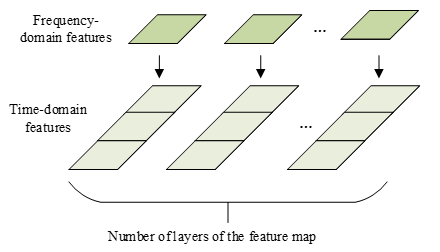Figure 1. Feature splicing layer

Firstly, the frequency-domain features extracted from the original echo signals received by local radar stations are copied based on the number of channels in the feature map outputted from the spliced hidden layer. Next, the copied features corresponding to a channel are attached to the end of the original hidden layer feature map. The feature map of the serial frequency-domain features of the new echo signals is then imported to the next layer of the network.

The cross-entropy loss of the network can be expressed as:

$CEL=-\frac{1}{M}\sum\limits_{a}{\left[ bln\beta +\left( 1-b \right)ln\left( 1-\beta \right) \right]}$     (20)

Let a and M be the number of classes of radar signals, and the number of samples in the test set of original echo signals, respectively; b be the number of positive samples; β be the number of samples predicted as positive by the classifier.

Our network needs to be trained in two stages: the training of the network except the splicing layer, and the training of the entire network. Let CV be the estimated importance of the frequency-domain features of echo signals; SU1 and SU2 be the losses of the original CNN in stage 1 and stage 2, respectively. For the feature map outputted by CP3, the error matrix before the addition of the splicing layer differs from that after the addition. The difference can be computed by a 2-norm ||R1-R2||. After the addition of the splicing layer, the error matrix of the frequency-domain features for the echo signals can be expressed as ||G2||2. The importance of the frequency-domain features for the echo signals can be calculated by:

$C{{V}_{d}}=\frac{S{{U}_{1}}-S{{U}_{2}}}{S{{U}_{2}}}\left( \left\| {{R}_{1}}-{{R}_{2}} \right\|+{{\left\| {{G}_{2}} \right\|}_{2}} \right)$     (21)

Formula (25) shows that the characteristic error of the splicing layer and the value of the cross-entropy loss function are positively correlated with the frequency-domain eigenvalue of the echo signals, while SU2 is negatively correlated with the frequency-domain eigenvalue of the echo signals. Let k be the serial number of network layers; e be a node on the current layer; ξ be the error matrix of the feature map of the current layer; ε' be the derivative of the activation function; US be the up-sampling operation; $\oplus$ be the Hadamard product. The error matrix of formula (25) can be obtained by combining formulas (26)-(28). For each convolutional layer:

${{\xi }^{k-1}}={{\xi }^{l}}\frac{\partial {{c}^{k}}}{\partial {{c}^{k-1}}}$     (22)

$\frac{\partial {{c}^{k}}}{\partial {{c}^{k-1}}}={{\xi }^{k}}*rot180\left( {{\theta }^{k}} \right)\oplus \varepsilon '\left( {{c}^{k-1}}-1 \right)$      (23)

For each pooling layer:

${{\xi }^{k-1}}=US\left( {{\xi }^{k}} \right)\oplus \varepsilon '\left( {{c}^{k}} \right)$     (24)

After computing the importance of frequency-domain features for the echo signals, the recognition algorithm for marine distributed radar signals, which fuse time-frequency features, can be designed further based on the CNN. Based on the calculation results of the above parameters, the network structure was determined according to the weighted fusion detection results for heterogenous radar signals. The flow of the complete algorithm is illustrated in Figure 2.

## 2.png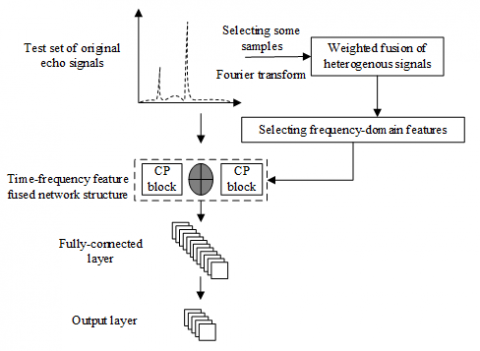Figure 2. Flow of distributed radar signal recognition algorithm

5. Experiments and Results Analysis

## 3.png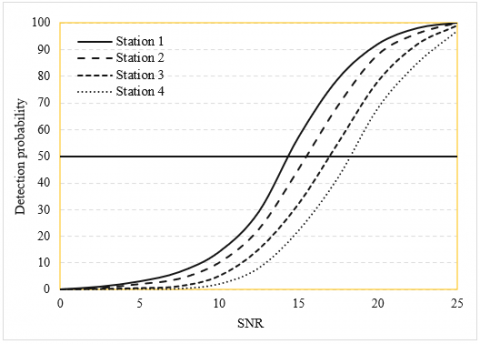Figure 3. Weights of signals received by different radar stations under unknown SNRs

Under unknown SNRs, the expected detection probability was set to 50%. Then, the weights of signals received by four local radar stations in the marine distributed radar system were plotted (Figure 3). Then, the proposed weighted fusion algorithm for heterogenous signals of marine distributed radars was applied, and the detection performance of the algorithm was analyzed.

## 4.png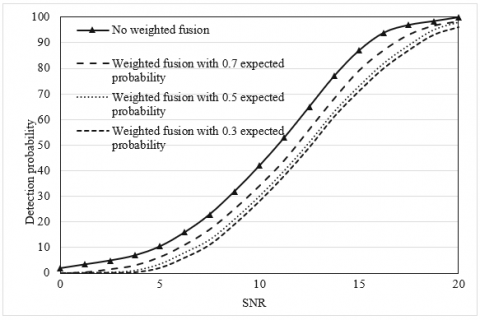Figure 4. Performance of weighted fusion algorithm vs. performance of original fusion algorithm

Figure 4 compares the performance of weighted fusion algorithm and that of original fusion algorithm. Table 2 presents the relationship between weight and expected detection probability under three different cases: In Case 1, there are 7, 12, 17, and 22 reference units; In Case 2, there are 7, 14, 21, and 28 reference units; In Case 3, there are 28, 24, 20, and 16 units.

Table 2. Relationship between weight and expected detection probability under different number of reference units

 Weight 0.7 0.5 0.3 Case 1 ω1 0.6858 0.6715 0.6824 ω2 0.8526 0.8547 0.8632 ω3 0.9254 0.8946 0.9214 ω4 1.002 1.023 1.025 Case 2 ω1 0.6254 0.6345 0.6285 ω2 0.8462 0.8512 0.8647 ω3 0.9548 0.9521 0.9648 ω4 1.004 1.002 1.006 Case 3 ω1 1.002 1.005 1.003 ω2 0.8457 0.8596 0.871 ω3 0.6528 0.6413 0.6625 ω4 0.4625 0.4749 0.5213

The above simulation results show that our weighted fusion algorithm outperformed the approaches without weighted fusion. Besides, the weighted fusion performance was not very different between the expected probabilities of 0.3, 0.5, and 0.7, suggesting the high stability of our weighted fusion algorithm. As the expected probability increased to 0.3, 0.5, and 0.7, the weighted fusion performance was 0.3, 0.31 and 0.29dB better in SNR than the performance of the original fusion algorithm, respectively. According to the algorithm performance curves at 7 and 28 reference units, the algorithm did not surpass the upper or lower bound of detection performance, which helps to measure the maximum degree of improvement of our algorithm against the original algorithm. As shown in Figure 4, the weighted fusion in Case 3 with the detection probability of 50% had an SNR gain of 0.9dB against the original fusion algorithm in other cases. Hence, the proposed algorithm can improve the performance by a maximum of 30%.

## 5.png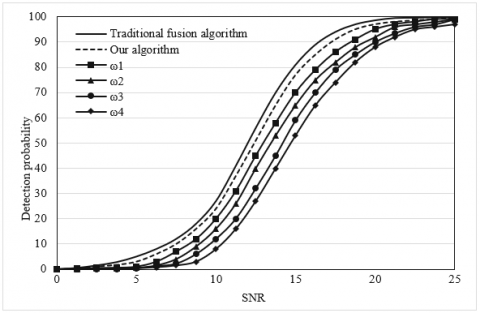Figure 5. Algorithm performance curves when the SNR satisfies certain conditions

Figure 5 shows the algorithm performance curves when the SNR satisfies certain conditions. Under most weighting methods, the weighted fusion algorithm outshined the traditional fusion algorithm. When the SNR satisfied XZB1=XZB2-4=XZB3-4, the third type of weight for the signals received by local radar stations would deteriorate. Compared with the SNR required for original fusion, the weighting with the third type of weight at the detection probability of 50% led to a 0.4dB higher SNR. The performance was good in all the other cases.

## 61.png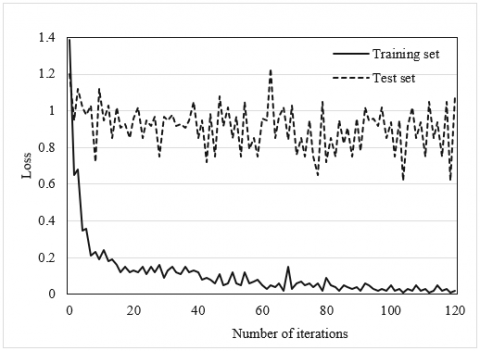(1) Loss curve

## 62.png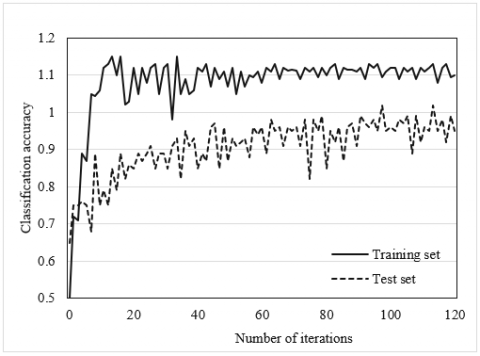(2) Accuracy curve

Figure 6. Training curves of the original CNN

## 71.png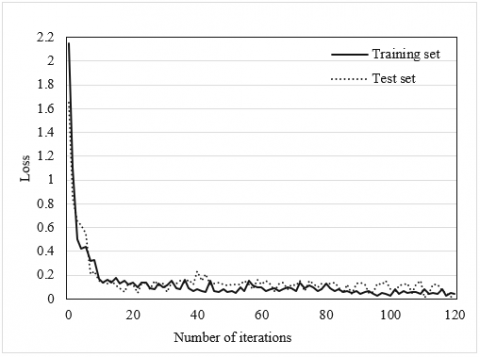(1) Loss curve

## 72.png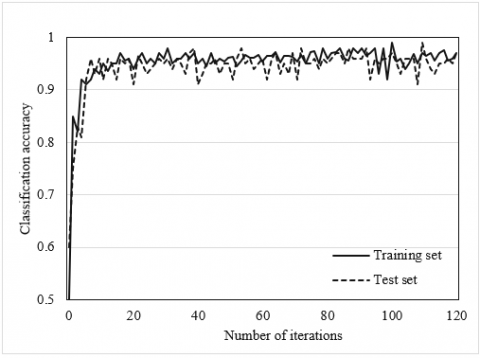(2) Accuracy curve

Figure 7. Training curves of the improved CNN

Figures 6 and 7 display the training curves of the original network (without the splicing layer) and the improved network (with the splicing layer), respectively. The curves of both networks tended to be stable after 60 iterations. However, the recognition error of the original network on the test set oscillated, while the improved network saw a steadily decreasing error and converged rapidly.

The comparison between Figures 6 and 7 shows that frequency-domain features effectively suppress network overfitting, and improve the recognition accuracy of marine distributed radar signals. This is because our network focuses on the frequency-domain parametric features that positively affect network decision. The screened time- and frequency-domain features are spliced on the splicing layer. Hence, compared with time-domain feature-based recognition algorithm, our algorithm improves the generalization ability and recognition accuracy of the detection model.

Figure 8 compares the recognition effects of different algorithms under the same datasets. The algorithms include our algorithm 1, the LSTM 2, the traditional recurrent neural network (RNN) 3, and the traditional CNN 4. Datasets 1 and 2 were collected by similar approaches from distributed radar systems in different sea areas. The two datasets cover basically the same types of signals. But Dataset 1 is 1.5 times that of Dataset 2. Both datasets were divided into a training set and a test set by the same split ratio. The classification accuracy of radar signals is the mean of the results of 150 signal recognition experiments. It can be seen that the recognition accuracy on Dataset 2 was higher than that on Dataset 1.

## 8.png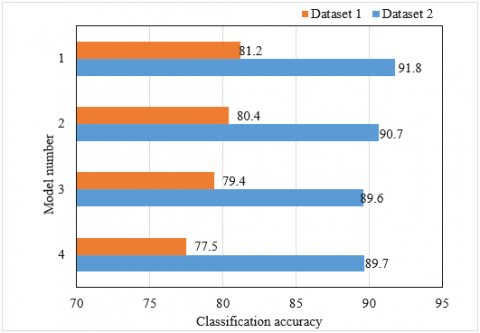Figure 8. Recognition effects of different algorithms under the same datasets

To compare denoising performance, the recognition effects of the four algorithms were compared under different noise levels (Figure 9). As the SNR changed from 0dB to 25dB, our algorithm achieved a much higher recognition accuracy than the other algorithms under a high SNR, reaching around 0.95.

## 9.png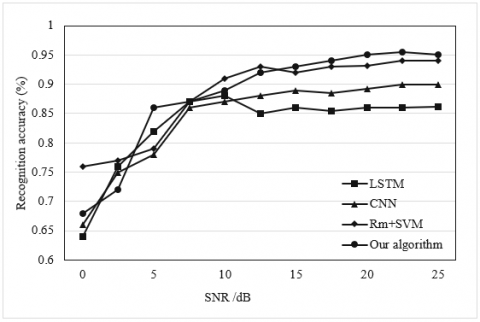Figure 9. Recognition effects of four algorithm under different noise levels

6. Conclusions

This paper recognizes and classifies marine distributed radar signals based on an improved deep neural network. Specifically, the authors gave a method for extracting the time-frequency features of distributed radar signals, proposed a weighted fusion detection algorithm for the heterogenous signals of marine distributed radars, and detailed the calculation of four types of weights. Finally, a CNN with feature splicing operation was established, the frequency-domain priori model feature assistive training was introduced to train the traditional DCNN, and the time- and frequency-domain signals were combined as the basis for classifying radar signals. Through experiments, our weighted fusion algorithm was compared with the original fusion algorithm in terms of the performance and the relationship between weight and expected detection probability, under different number of reference units. The comparison shows that our weighted fusion algorithm outperforms the fusion algorithm without weighted fusion. In addition, the training curves of the original network (without splicing layer) were compared with the improved network (with splicing layer), indicating that the improved network saw a steadily decreasing error and converged rapidly. Finally, the recognition effects of different algorithms were compared under the same datasets and different noise levels. The proposed algorithm was found to be superior and effective.

Acknowledgment

This article are supported by the project of Guangdong Provincial Science and Technology Department's subsidy for people's livelihood in 2020 and other institutional development expenditure funds (overseas famous teachers, 2020A1414010380), by the project of 2021 Guangdong Province Science and Technology Special Funds (‘College Special Project + Task List’) Competitive Distribution (2021A501-11), by the project of Enhancing School with Innovation of Guangdong Ocean University’s (230420023), and by the program for scientific research start-up funds of Guangdong Ocean University (R20065).

References

 Sola, I., Fernández-Torquemada, Y., Forcada, A., Valle, C., del Pilar-Ruso, Y., González-Correa, J.M., Sánchez-Lizaso, J.L. (2020). Sustainable desalination: Long-term monitoring of brine discharge in the marine environment. Marine Pollution Bulletin, 161: 111813. https://doi.org/10.1016/j.marpolbul.2020.111813

 Lee, C., Kim, H.R. (2019). Conceptual development of sensing module applied to autonomous radiation monitoring system for marine environment. IEEE Sensors Journal, 19(19): 8920-8928. https://doi.org/10.1109/JSEN.2019.2921550

 Li, Z., Jin, Z.Q., Shao, S.S., Xu, X. (2018). A review on reinforcement corrosion mechanics and monitoring techniques in concrete in marine environment. Mater. Rev. A Rev. Pap, 32: 4170-4181.

 Wang, X.H., Ma, R., Cao, X., Cao, L., Chu, D.Z., Zhang, L., Zhang, T.P. (2017). Software for marine ecological environment comprehensive monitoring system based on MCGS. In IOP Conference Series: Earth and Environmental Science, 82(1): 012087. https://doi.org/10.1088/1755-1315/82/1/012087

 Min, R., Liu, Z., Pereira, L., Yang, C., Sui, Q., Marques, C. (2021). Optical fiber sensing for marine environment and marine structural health monitoring: A review. Optics & Laser Technology, 140: 107082. https://doi.org/10.1016/j.optlastec.2021.107082

 Branchet, P., Arpin-Pont, L., Piram, A., Boissery, P., Wong-Wah-Chung, P., Doumenq, P. (2021). Pharmaceuticals in the marine environment: What are the present challenges in their monitoring. Science of The Total Environment, 766: 142644. https://doi.org/10.1016/j.scitotenv.2020.142644

 Liu, G., Rui, G., Tian, W., Wu, L., Cui, T., Huang, J. (2021). Compressed sensing of 3D marine environment monitoring data based on spatiotemporal correlation. IEEE Access, 9: 32634-32649. https://doi.org/10.1109/ACCESS.2021.3060472

 Zhu, Y., Han, Y. (2021). Marine environment monitoring based on virtual reality and fuzzy C-means clustering algorithm. Mobile Information Systems, 2021: Article ID 2576919. https://doi.org/10.1155/2021/2576919

 Beltrán-Sanahuja, A., Casado-Coy, N., Simó-Cabrera, L., Sanz-Lázaro, C. (2020). Monitoring polymer degradation under different conditions in the marine environment. Environmental Pollution, 259: 113836. https://doi.org/10.1016/j.envpol.2019.113836

 Du, Y., Yin, J., Tan, S., Wang, J., Yang, J. (2020). A numerical study of roughness scale effects on ocean radar scattering using the second-order SSA and the moment method. IEEE Transactions on Geoscience and Remote Sensing, 58(10): 6874-6887. https://doi.org/10.1109/TGRS.2020.2977368

 Wyatt, L.R. (2019). Measuring the ocean wave directional spectrum ‘First Five’ with HF radar. Ocean Dynamics, 69(1): 123-144. https://doi.org/10.1007/s10236-018-1235-8

 Zhao, X.B., Yan, W., Ai, W.H., Lu, W., Ma, S. (2019). Research on calculation method of Doppler centroid shift from airborne synthetic aperture radar for ocean feature retrieval. Journal of Radars, 8(3): 391-399. https://doi.org/10.12000/JR19020

 Cosoli, S., Grcic, B., De Vos, S., Hetzel, Y. (2018). Improving data quality for the Australian high frequency ocean radar network through real-time and delayed-mode quality-control procedures. Remote Sensing, 10(9): 1476. https://doi.org/10.3390/rs10091476

 Lu, Y., Zhang, B., Perrie, W., Mouche, A., Zhang, G. (2020). CMODH validation for C-band synthetic aperture radar HH polarization wind retrieval over the ocean. IEEE Geoscience and Remote Sensing Letters, 18(1): 102-106. https://doi.org/10.1109/LGRS.2020.2967811

 Naz, S., Iqbal, M.F., Mahmood, I., Allam, M. (2021). Marine oil spill detection using Synthetic Aperture Radar over Indian Ocean. Marine Pollution Bulletin, 162: 111921. https://doi.org/10.1016/j.marpolbul.2020.111921

 Yao, G., Xie, J., Huang, W. (2020). HF radar ocean surface cross section for the case of floating platform incorporating a six-DOF oscillation motion model. IEEE Journal of Oceanic Engineering, 46(1): 156-171. https://doi.org/10.1109/JOE.2019.2959289

 Ren, L., Chu, N., Hu, Z., Hartnett, M. (2020). Investigations into synoptic spatiotemporal characteristics of coastal upper ocean circulation using high frequency radar data and model output. Remote Sensing, 12(17): 2841. https://doi.org/10.3390/rs12172841

 Liu, X., Cui, S., Zhao, C., Wang, P., Zhang, R. (2018). Bind intra-pulse modulation recognition based on machine learning in radar signal processing. In International Conference in Communications, Signal Processing, and Systems, pp. 717-729. https://doi.org/10.1007/978-981-13-6504-1_87

 Yi, J.D., Yang, J. (2020). Radar signal recognition based on IFOA-SA-BP neural network. Systems Engineering and Electronics, 42(12): 2735-2741. https://doi.org/10.3969/j.issn.1001-506X.2020.12.08

 Shi, L.M., Yang, C.Z., Wu, H.C. (2020). Radar signal recognition method based on deep residual network and triplet loss. Systems Engineering and Electronics, 42(11): 2506-2512. https://doi.org/10.3969/j.issn.1001-506X.2020.11.12

 Bai, J., Gao, L., Gao, J., Li, H., Zhang, R., Lu, Y. (2019). A new radar signal modulation recognition algorithm based on time-frequency transform. In 2019 IEEE 4th International Conference on Signal and Image Processing (ICSIP), pp. 21-25. https://doi.org/10.1109/SIPROCESS.2019.8868675

 Gao, L., Zhang, X., Gao, J., You, S. (2019). Fusion image based radar signal feature extraction and modulation recognition. IEEE Access, 7: 13135-13148. https://doi.org/10.1109/ACCESS.2019.2892526

 Liu, B., Feng, Y., Yin, Z., Fan, X. (2019). Radar signal emitter recognition based on combined ensemble empirical mode decomposition and the generalized S-transform. Mathematical Problems in Engineering, 2019: Article ID 2739173. https://doi.org/10.1155/2019/2739173

 Gao, J., Lu, Y., Qi, J., Shen, L. (2019). A radar signal recognition system based on non-negative matrix factorization network and improved artificial bee colony algorithm. IEEE Access, 7: 117612-117626. https://doi.org/10.1109/ACCESS.2019.2936669

 Qu, Z., Hou, C., Hou, C., Wang, W. (2020). Radar signal intra-pulse modulation recognition based on convolutional neural network and deep Q-learning network. IEEE Access, 8: 49125-49136. https://doi.org/10.1109/ACCESS.2020.2980363

 Li, D., Yang, R., Li, X., Zhu, S. (2020). Radar signal modulation recognition based on deep joint learning. IEEE Access, 8: 48515-48528. https://doi.org/10.1109/ACCESS.2020.2978875

 Wu, B., Yuan, S., Li, P., Jing, Z., Huang, S., Zhao, Y. (2020). Radar emitter signal recognition based on one-dimensional convolutional neural network with attention mechanism. Sensors, 20(21): 6350. https://doi.org/10.3390/s20216350

 Wei, S., Qu, Q., Zeng, X., Liang, J., Shi, J., Zhang, X. (2021). Self-attention Bi-LSTM networks for radar signal modulation recognition. IEEE Transactions on Microwave Theory and Techniques, 69(11): 5160-5172. https://doi.org/10.1109/TMTT.2021.3112199

 Liu, L., Li, X. (2021). Radar signal recognition based on triplet convolutional neural network. EURASIP Journal on Advances in Signal Processing, 2021(1): 1-16. https://doi.org/10.1186/s13634-021-00821-8

 Wang, Y., Cao, G., Su, D., Wang, H., Ren, H. (2021). Embedding bottleneck gated recurrent unit network for radar signal recognition. In 2021 International Joint Conference on Neural Networks (IJCNN), pp. 1-8. https://doi.org/10.1109/IJCNN52387.2021.9533995